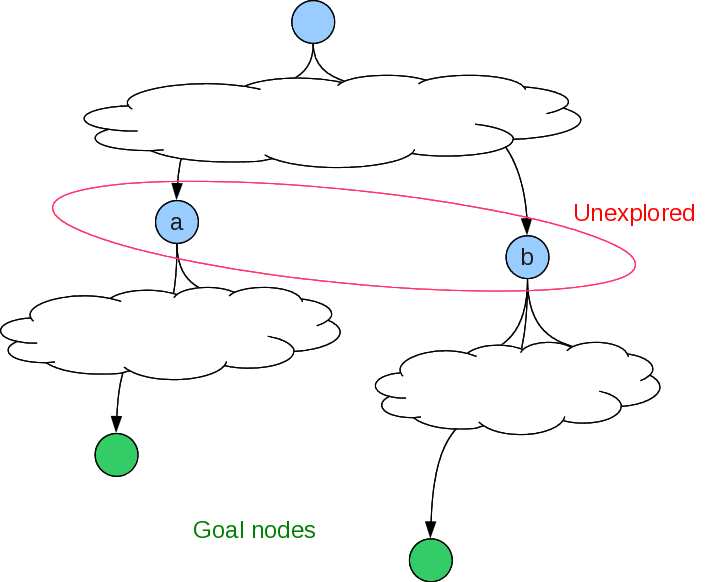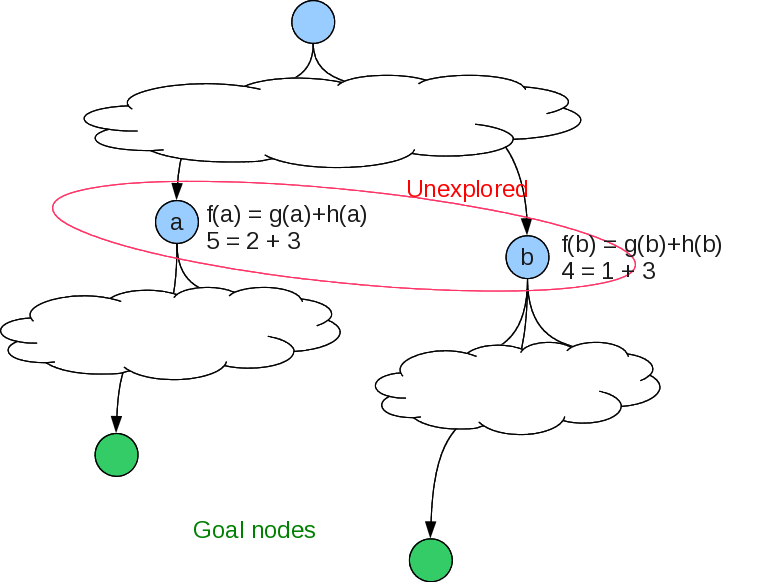# Informed Search¶

Also known as "heuristic" search, because the search is informed by an estimate of the total path cost through each node, and the next unexpanded node with the lowest estimated cost is expanded next.

At some intermediate node, the
estimated cost of the solution path =
the sum of the step costs so far from the start node to this node
+
an estimate of the sum of the remaining step costs to a goal



Let's label these as

• $f(n) =$ estimated cost of the solution path through node $n$
• $g(n) =$ the sum of the step costs so far from the start node to this node
• $h(n) =$ an estimate of the sum of the remaining step costs to a goal

heuristic function: $h(n) =$ estimated cost of the cheapest path from state at node $n$ to a goal state.Should we explore under Node a or b?# A* algorithm¶

## Non-recursive¶

So, now you know enough python to try to implement A*, at least a non-recursive form. Start with your graph search algorithm from Assignment 1. Modify it so that the next node selected is based on its f value.

For a given problem, define start_state, actions_f, take_action_f, goal_test_f, and a heuristic function heuristic_f. actions_f must return valid actions paired with the single step cost, and take_action_f must return the pair containing the new state and the cost of the single step given by the action. We can use the Node class to hold instances of nodes. However, since this is not a recursive algorithm, Node must be extended to include the node's parent node, to be able to generate the solution path once the search finds the goal.

Now the A* algorithm can be written as follows

• Initialize expanded to be an empty dictionary
• Initialize un_expanded to be a list containing the start_state node. Its h value is calculated using heuristic_f, its g value is 0, and its f value is g+h.
• If start_state is the goal_state, return the list containing just start_state and its f value to show the cost of the solution path.
• Repeat the following steps while un_expanded is not empty:
• Pop from the front of un_expanded to get the best (lowest f value) node to expand.
• Generate the children of this node.
• Update the g value of each child by adding the action's single step cost to this node's g value.
• Calculate heuristic_f of each child.
• Set f = g + h of each child.
• Add the node to the expanded dictionary, indexed by its state.
• Remove from children any nodes that are already either in expanded or un_expanded, unless the node in children has a lower f value.
• If goal_state is in children:
• Build the solution path as a list starting with goal_state.
• Use the parent stored with each node in the expanded dictionary to construct the path.
• Reverse the solution path list and return it.
• Insert the modified children list into the un_expanded list and sort by f values.

## Recursive¶

Our authors provide the Recursive Best-First Search algorithm, which is A* in a recursive, iterative-deepening form, where depth is now given by the $f$ value. Other differences from just iterative-deepening A* are:

• depth-limit determined by $f$ value of best alternative to node being explored, so will stop when alternative at the node's level looks better;
• $f$ value of a node is replaced by best $f$ value of its children, so any future decision to try expanding this node again is more informed.

It is a bit difficult to translate their pseudo-code into python. Here is my version. Let's step through it.

In :
%%writefile a_star_search.py
# Recursive Best First Search (Figure 3.26, Russell and Norvig)
#  Recursive Iterative Deepening form of A*, where depth is replaced by f(n)

class Node:

def __init__(self, state, f=0, g=0, h=0):
self.state = state
self.f = f
self.g = g
self.h = h

def __repr__(self):
return f'Node({self.state}, f={self.f}, g={self.g}, h={self.h})'

def a_star_search(start_state, actions_f, take_action_f, goal_test_f, heuristic_f):
h = heuristic_f(start_state)
start_node = Node(state=start_state, f=0 + h, g=0, h=h)
return a_star_search_helper(start_node, actions_f, take_action_f,
goal_test_f, heuristic_f, float('inf'))

def a_star_search_helper(parent_node, actions_f, take_action_f,
goal_test_f, heuristic_f, f_max):

if goal_test_f(parent_node.state):
return ([parent_node.state], parent_node.g)

## Construct list of children nodes with f, g, and h values
actions = actions_f(parent_node.state)
if not actions:
return ('failure', float('inf'))

children = []
for action in actions:
(child_state, step_cost) = take_action_f(parent_node.state, action)
h = heuristic_f(child_state)
g = parent_node.g + step_cost
f = max(h + g, parent_node.f)
child_node = Node(state=child_state, f=f, g=g, h=h)
children.append(child_node)

while True:
# find best child
children.sort(key = lambda n: n.f) # sort by f value
best_child = children
if best_child.f > f_max:
return ('failure', best_child.f)
# next lowest f value
alternative_f = children.f if len(children) > 1 else float('inf')
# expand best child, reassign its f value to be returned value
result, best_child.f = a_star_search_helper(best_child, actions_f,
take_action_f, goal_test_f,
heuristic_f,
min(f_max,alternative_f))
if result != 'failure':                    #        g
result.insert(0, parent_node.state)    #       /
return (result, best_child.f)          #      d
#     / \
if __name__ == "__main__":                         #    b   h
#   / \
successors = {'a': ['b','c'],                  #  a   e
'b': ['d','e'],                  #   \
'c': ['f'],                      #    c   i
'd': ['g', 'h'],                 #     \ /
'f': ['i','j']}                  #      f
#      \
def actions_f(state):                          #       j
try:
## step cost of each action is 1
return [(succ, 1) for succ in successors[state]]
except KeyError:
return []

def take_action_f(state, action):
return action

def goal_test_f(state):
return state == goal

def h1(state):
return 0

start = 'a'
goal = 'h'
result = a_star_search(start, actions_f, take_action_f, goal_test_f, h1)

print(f'Path from a to h is {result} for a cost of {result}')

Overwriting a_star_search.py


Running this shows

In :
run a_star_search.py

Path from a to h is ['a', 'b', 'd', 'h'] for a cost of 3

In :
actions_f('a')
valid_ones = actions_f('a')
valid_ones

Out:
[('b', 1), ('c', 1)]
In :
take_action_f('a', valid_ones)

Out:
('b', 1)

Actually, there is in error in this code. Try using it to search for a goal that does not exist!